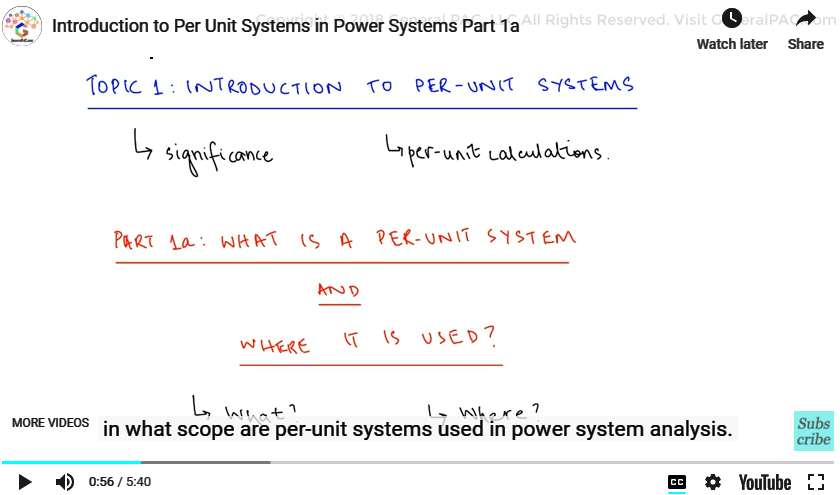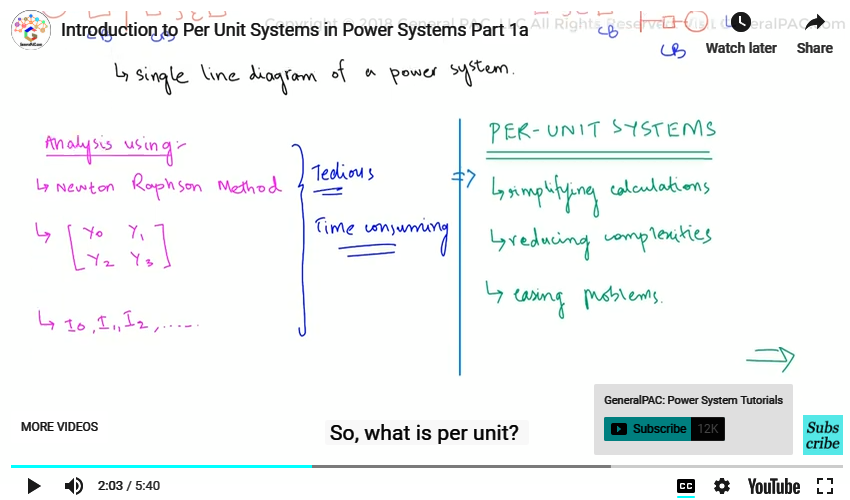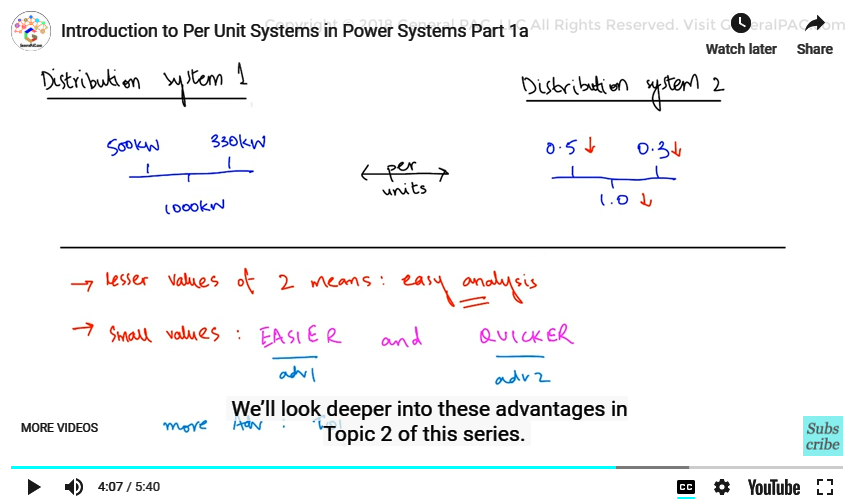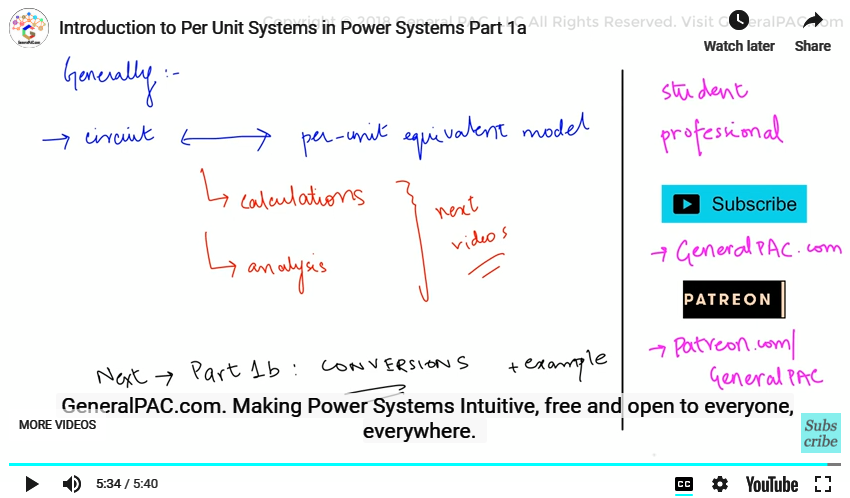This video was brought to you by GeneralPAC.com, making power systems Intuitive, Open and Free for Everyone, Everywhere. Consider subscribing and supporting through patreon.com/GeneralPAC. This is a mechanism for you to support us financially so we can continue making high quality power system video tutorials. Our corporate sponsor for this topic is AllumiaX.com from Seattle, Washington. Contact them for industrial and commercial power system studies.

Introduction to Per-Unit Systems. This is the first part of the series called “Introduction to Per-Unit Systems”. And in this series, we will go over the significance of per unit systems in power system analysis that will help us intuitively understand the per-unit calculations involved.

In this video, Part 1a, we will be discussing what per unit systems are and in what scope are per-unit systems used in power system analysis.So, we know that power systems consists of many components like generators, transformers, loads, protective devices, capacitor banks, reactors and so on. In order to analyze these devices and circuits that make up the power systems, we use many tools and techniques like Newton Raphson method, building the admittance impedance matrices, performing iterative calculations and all of these analysis methodologies are tedious calculations and consumes quite a bit of time. But they can be more simplified by incorporating per unit systems.

So basically per-unit systems and per-unit calculations are used in aid to simplifying the mathematical calculations as well as reducing the theoretical complexities of power system analysis. And power systems are easier to solve and they’re easier to comprehend with per units, and that’s precisely what we are going to demonstrate in some of the upcoming videos in this series.

So, what is per unit? A per unit system is any quantity that is taken with respect to a defined unit value. And so, a per unit system is defined as a power system that has its quantities defined against a unit value, and we usually refer this unit value as the base value.For example, consider taking a quantity of 15 with respect to a defined value of 100, the per unit quantity, taken as the ratio of the original against the defined value will be 0.15 per unit. In Power Systems Engineering this per unit value can take a form of voltages, currents, impedances and power ratings.

Now, since per unit is a fraction, made as a result of dividing by a base, this means they are smaller, then that per unit systems that contain smaller values compared to a power system containing the actual value, which are bigger in magnitude. So, for example, consider a distribution system that has a load like 500 kW, 1000 kW, 330 kW and so forth.

Now consider the same system converted to per-unit with loads like 0.5, 1.0 or 0.3. The lesser value represents that the analysis of the second system is much easier than the first one. So, the values are dropped down and they’re much easier to work with. Basically, that’s what we’re saying. Now, since the smaller values are easier to perform with calculations and so for the techniques is used to make analysis of power systems quicker and easier. And therefore, it has many advantages which is why we convert systems into per unit equivalents. We’ll look deeper into these advantages in Topic 2 of this series.So, coming up to the next point: where are per-unit values used? There are a lot of sections of a power system that need to be analyzed so where do we exactly employ per unit techniques? So, the per unit technique is used in a variety of Power Systems Engineering Analysis and calculations such as when we refer values of a transformer to primary or secondary side; analyze a system containing multiple voltage levels for example a combination of transformers, short circuit studies, load flow studies and in pretty much every other power system analysis technique.

Generally, any circuit can be converted into per units equivalent model using some calculations and some analysis that can be carried out. So, we’ll be explaining these calculations involved and how per unit systems can help in power system analysis in the upcoming videos. In the next video we will look at a general procedure that is employed to convert values to a per unit equivalent. So, we’ll give an example.

Now, we hope that you have a continued interest in this topic and series as a student or professional. And we also hope you find this content useful and enlightening. Please consider subscribing to GeneralPac.com and becoming our patron on patreon.com/GeneralPAC.

GeneralPAC.com. Making Power Systems Intuitive, free and open to everyone, everywhere. Thank you.#### Greetings from the GeneralPAC Team!

We make high-quality Power Systems Video Tutorials on complex topics that are free and open to everyone!  Thank you so much for supporting us through Patreon so we can continue doing good and valuable work.

What is Patreon and why do we use it?

Patreon is a fantastic portal that allows our fans and community to make monthly contribution (like Netflix subscription) so we can continue creating high-quality power systems video tutorials. In return, you get access to incredible perks like voting on future topics, getting your questions answered, access to VIP Q/A webinars with the creators of GeneralPAC, and much more! We THANK YOU for supporting us

Why do we need your support?

An incredible amount of time and effort is needed to develop high-quality video tutorials. Each video (Part 1 for example) takes approximately 10 hours to complete which includes learning the concept ourselves, brainstorming creative ways to teach and explain the concepts, writing the script, audio recording, video recording, and editing. It's no wonder why Hundreds-of-Thousands of people have watched, liked, subscribed, and left positive comments on Youtube channel. Your support truly makes all the difference.